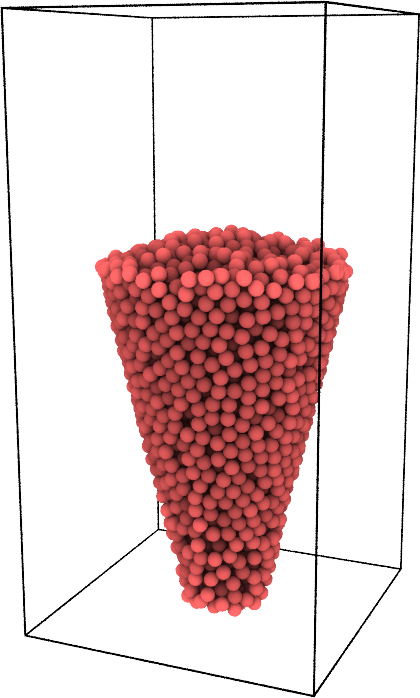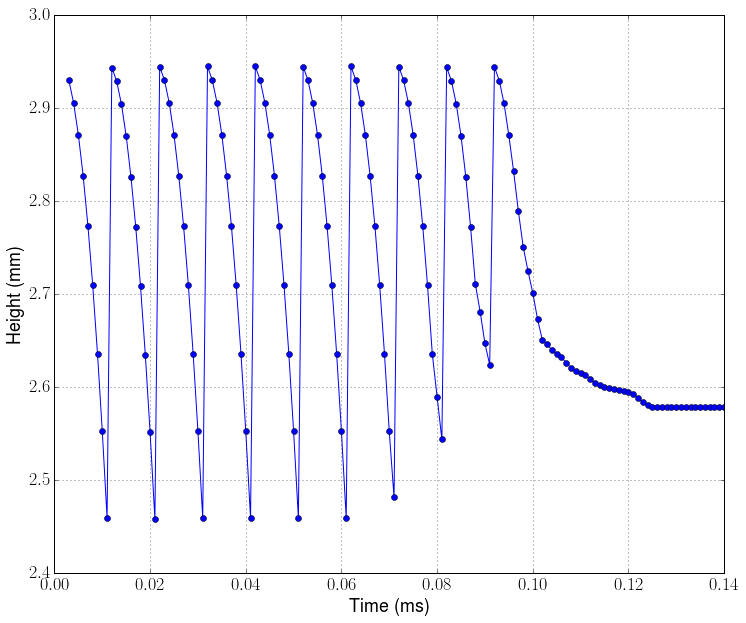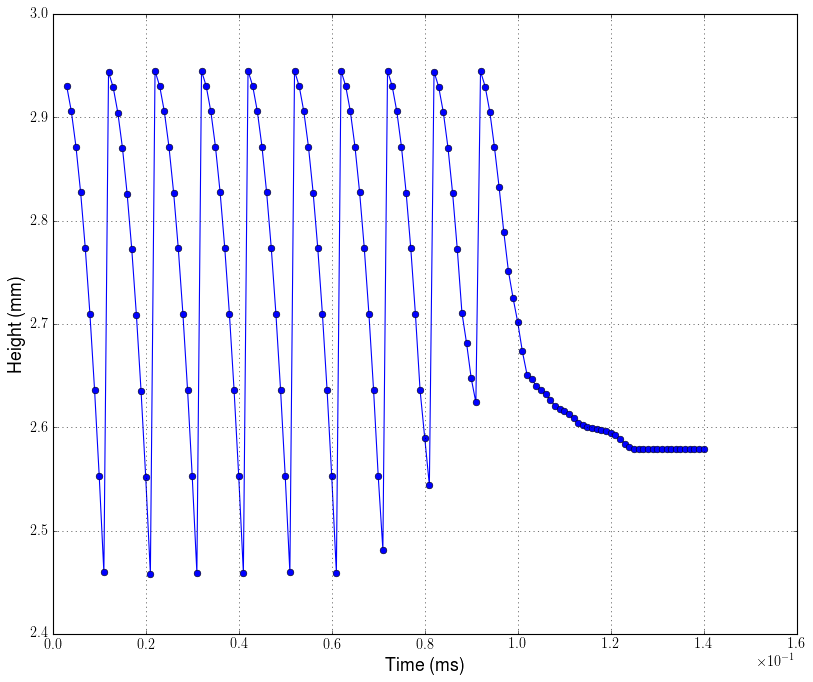# Temporal Analysis¶

In this tutorial, a PyGran System class is created from a LIGGGHTS trajectory dump file. This class is then used to plot different strctural variables as a function of time.

In :
# Import PyGran + matplotlib modules
from PyGran import analysis
import matplotlib.pylab as plt

# Create a PyGran System from a dump file (default si units)
System = analysis.System(Particles='traj*.dump')

# Timestep used in simulation (s)
dt = 1e-6

Out:# Bed Height¶

The code below computes the bed height (along the z-direction) and then plots it as a function of time using pylab.

In :
from numpy import array

# Skip empty frames
System.skip()

# Extract bed height + timesteps
data = array([[ts * dt,System.Particles.z.max()] for ts in System])

# Plot bed height (mm) vs time (ms)
plt.plot(data[:,0] * 1e3, data[:,1] * 1e3, '-o')# Height Bed (alternative method)¶

Alternatively, one can use PyGran's plot2D module as shown below.

In :
# Import plot2D module which uses matplotlib for generating 2D plots
from PyGran.plotter import plot2D

# timePlot is a method that plots an attribute (in this case z) as a function of time (scaled by dt).
# The default metric is the mean. Any numpy method can used (such as min, max, etc.) or a PyGran.Particles
# method, such as com, density, etc.
fig = plot2D.timePlot(System, attr='z', metric='max', figsize=(12,10), xscale=dt*1e3, yscale=1e3, \
lineStyle='-', marker='o')

Out:
<matplotlib.text.Text at 0x7fc32dbcb410>